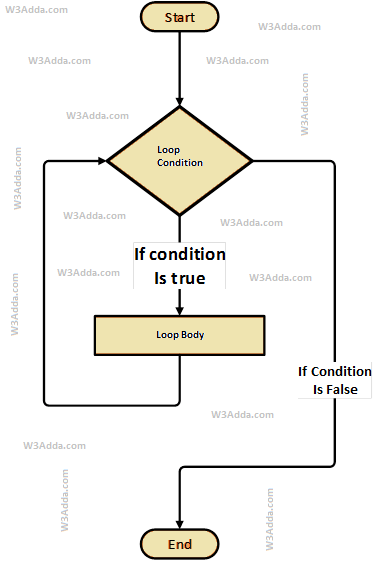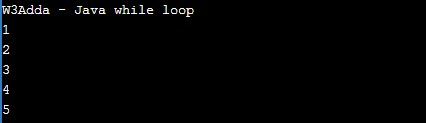# Java while Loop

In this tutorial you will learn about the Java while Loop and its application with practical example.

## Java while Loop

The while loop will execute a block of statements as long as a test expression is true. While loop in java is useful when the number of iterations can not be predicted beforehand. The while loop evaluates test expression at beginning of each pass.

## Java While Loop Flow DiagramSyntax:-

Here, a condition is a Boolean expression that results in either True or False, if it results in True then statements inside the loop body are executed and the condition is evaluated again. This process is repeated until the condition is evaluated as False. If the condition results in False then execution is skipped from the loop body and while loop is terminated.

Example:-

Here, we have initialized ctr and maxCtr to 1 and 5 respectively, in the next statement we have a while loop, that checks the condition ctr <= maxCtr for each iteration. If the test condition returns True, the statements inside the loop body are executed and if the test condition returns False then the while loop is terminated. Inside the loop body, we have incremented the ctr value by one for each iteration. When we run the above java program, we see the following output –

Output:-In this tutorial we have learn about the Java while Loop and its application with practical example. I hope you will like this tutorial.